# In the figure, four charges, given in multiples of 1.00×10-5 C form the corners of a...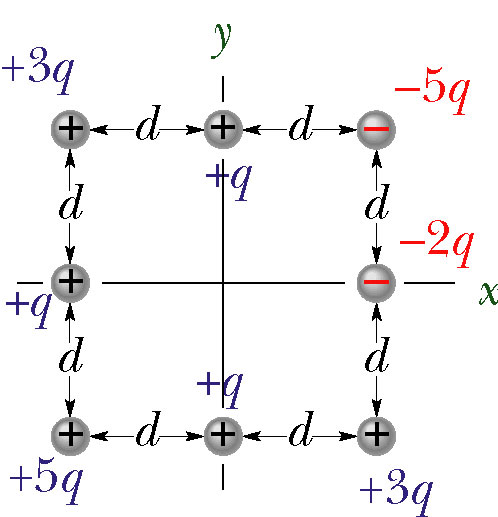In the figure, four charges, given in multiples of 1.00×10-5 C form the corners of a square and four more charges lie at the midpoints of the sides of the square. The distance between adjacent charges on the perimeter of the square is d = 5.90×10-2 m. What are the magnitude and direction of the electric field at the center of the square?

The magnitude of E? Ex? Ey?

bg+ bs+ D+ bgCp PH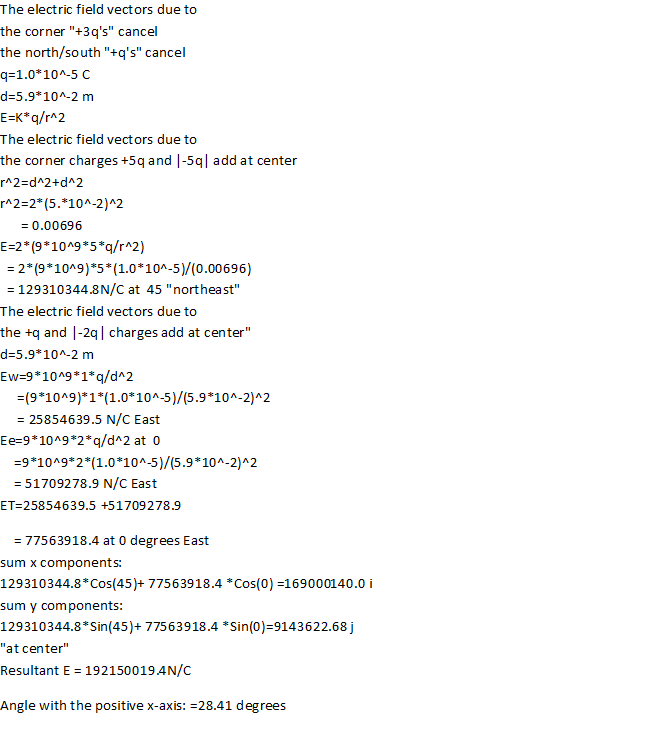#### Earn Coin

Coins can be redeemed for fabulous gifts.

Similar Homework Help Questions
• ### (c23p64) In the figure, four charges, given in multiples of 1.00×10-6 C form the corners of...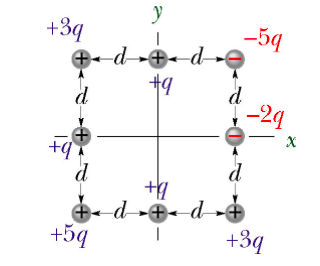(c23p64) In the figure, four charges, given in multiples of 1.00×10-6 C form the corners of a square and four more charges lie at the midpoints of the sides of the square. The distance between adjacent charges on the perimeter of the square is d = 2.40×10-2 m. a)What are the magnitude and direction of the electric field at the center of the square? The magnitude of E? b) What is the X component of the electric field (Ex) at...

• ### In the figure, four charges, given in multiples of 9.00×10-6 C form the corners of a...In the figure, four charges, given in multiples of 9.00×10-6 C form the corners of a square and four more charges lie at the midpoints of the sides of the square. The distance between adjacent charges on the perimeter of the square is d = 3.90×10-2 m. What are the magnitude and direction of the electric field at the center of the square? The magnitude of E, Ex, and Ey? 52| 1

• ### Problem #6: In the figure, four charges, given in multiples of 3.00×10-6 C form the corners...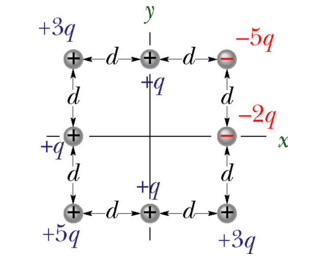Problem #6: In the figure, four charges, given in multiples of 3.00×10-6 C form the corners of a square and four more charges lie at the midpoints of the sides of the square. The distance between adjacent charges on the perimeter of the square is d = 7.00×10-3 m. What are the magnitude and direction of the electric field at the center of the square? 1) The magnitude of E? _______ 2) Ex? ________ 3) Ey? ________

• ### 8 ChargesIn the figure, four charges, given in multiples of 1.00×10-5 C form the corners of a square and four more charges lie at the midpoints of the sides of the square.The distance between adjacent charges on the perimeter of the square is d = 7.40×10-2 m. What are the magnitude and direction of the electric field at the centerof the square? The magnitude of E? Ex? Ey?

• ### Eight ChargesIn the figure, four charges, given in multiples of 1.00×10-5 C form the corners of a square and four more charges lie at the midpoints of the sides of the square.The distance between adjacent charges on the perimeter of the square is d = 7.40×10-2 m. What are the magnitude and direction of the electric field at the centerof the square? The magnitude of E? Ex? Ey?

• ### Four ChargesIn the figure, four charges, given in multiples of 8.00×10-6 C form the corners of a square and four more charges lie at themidpoints of the sides of the square. The distance between adjacent charges on the perimeter of the square is d = 3.00×10-2 m. What arethe magnitude and direction of the electric field at the center of the square? The magnitude of E? Ex?Ey?

• ### 8 Charges On a Sqare---will rate lifesaverIn the figure, four charges, given in multiples of8.00×10-6 C form the corners of a squareand four more charges lie at the midpoints ofthe sides of thesquare. The distance between adjacent charges on the perimeter ofthe square is d = 1.30×10-2 m. What arethe magnitude anddirection of the electric field at the center ofthe square? The magnitude of E?Ex?Ey?

• ### 8 Charges on Square

In the figure, four charges, given in multiples of 1.00×10-5 C form the corners of a square and four more charges lie at the midpoints of the sides of the square. Thedistance between adjacent charges on the perimeter of the square is d = 4.90×10-2 m. What are the magnitude and direction of the electric field at the center of thesquare? The magnitude of E?

• ### In the figure, four point charges lie on the corners of a square, with a fifth...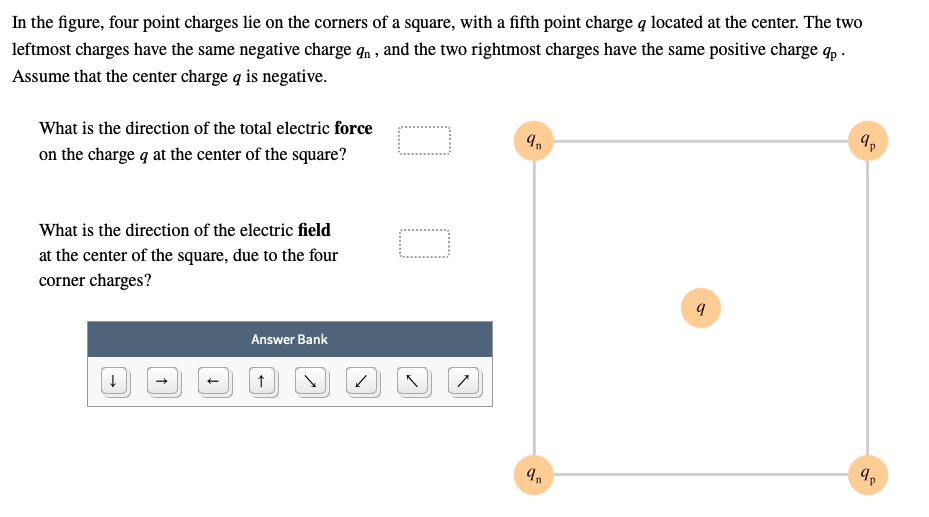In the figure, four point charges lie on the corners of a square, with a fifth point charge q located at the center. The two leftmost charges have the same negative charge qn, and the two rightmost charges have the same positive charge qp Assume that the center charge q is negative. What is the direction of the total electric force.... on the charge q at the center of the square? What is the direction of the electric field at...

• ### In the figure, four point charges lie on the corners of a square, with a fifth...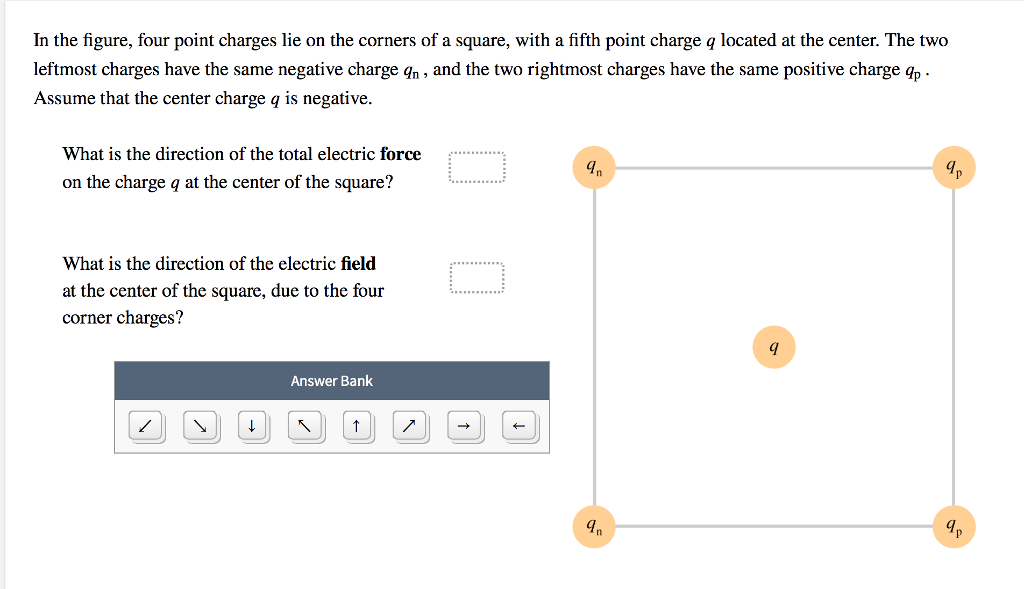In the figure, four point charges lie on the corners of a square, with a fifth point charge q located at the center. The two leftmost charges have the same negative charge qn , and the two rightmost charges have the same positive charge Assume that the center charge q is negative. What is the direction of the total electric force In on the charge q at the center of the square? What is the direction of the electric field...